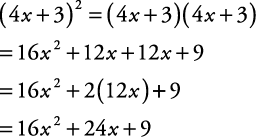## Special Products of Binomials

Two binomials with the same two terms but opposite signs separating the terms are called conjugates of each other. Following are examples of conjugates:##### Example 1

Find the product of the following conjugates.

1. (3 x + 2)(3 x – 2)

2. (–5 a – 4 b)(–5 a + 4 b)

1.2.Notice that when conjugates are multiplied together, the answer is the difference of the squares of the terms in the original binomials.

The product of conjugates produces a special pattern referred to as a difference of squares. In general,

( x + y)( xy) = x 2y 2

The squaring of a binomial also produces a special pattern.

##### Example 2

Simplify each of the following.

1. (4 x + 3) 2

2. (6 a – 7 b) 2

1.2.First, notice that the answers are trinomials. Second, notice that there is a pattern in the terms:

1. The first and last terms are the squares of the first and last terms of the binomial.

2. The middle term is twice the product of the two terms in the binomial.

The pattern produced by squaring a binomial is referred to as a square trinomial. In general,##### Example 3

Do the following special binomial products mentally.

1. (3 x + 4 y) 2

2. (6 x + 11)(6 x – 11)

1. (3 x + 4 y) 2 = 9 x 2 + 24 xy + 16 y 2

2. (6 x + 11)(6 x – 11) = 36 x 2 – 121